/
Features

# Sponsored Feature: Fluid Simulation for Video Games (Part 1)

This sponsored feature, part of Intel's Visual Computing site and written by Dr. Michael J. Gourlay of the University of Central Florida Interactive Entertainment Academy, begins a multi-part series that explains fluid dynamics and its simulation techniques.

[This sponsored feature, part of Intel's Visual Computing site and written by Dr. Michael J. Gourlay of the University of Central Florida Interactive Entertainment Academy, begins a multi-part series that explains fluid dynamics and its simulation techniques.]

### Introduction to Fluid Dynamics

Video games appeal to our desire to explore and interact with our environment, and adding real-world phenomena-such as fluid motion-allows game developers to create immersive and fun virtual worlds. Recently, physical simulations have become more realistic, but the simulations have largely been limited to rigid bodies.

Pervasive simulations of continuous media like cloth and fluids remain uncommon, largely because fluid dynamics entail conceptual and computational challenges that make simulating fluids difficult. This article begins a three-part series that explains fluid dynamics and its simulation techniques. The article culminates in an example of a fluid simulation algorithm suitable for use in a video game.

To get started in fluid simulations, you need to understand the fundamentals of fluid dynamics. Let's start by covering some of the basics.

## What Is a Fluid?

A fluid is any substance that flows (in other words, a substance that can take the shape of its container) and does not resist deformation (meaning that it slides when dragged). People often use fluid and liquid interchangeable, but technically, the term fluid can refer to either a liquid or a gas. A gas fills its container completely, whereas a liquid has a distinct "free surface" whose shape does not depend on its container. (Often, when you use computer graphics to visualize a liquid, you render only its surface-for example, ripples on a pond or a stream of water.) The distinction between liquids and gasses can influence how you model the fluid, but both obey the same basic fluid formulae and share similar properties.

But what about smoke? Smoke seems to behave like a gas but also appears to have a kind of surface, although perhaps not as distinct as that of a liquid. The answer is that smoke is really a combination of a gas and tiny suspended particulates, and the combination of these particulates is called an aerosol. Those particulates follow the motion of the gas (and let game players see that motion) without necessarily influencing the motion. You can usually treat smoke as a kind of gas, where one of its properties-for example, density or composition-varies.

## Varieties of Physical Simulation

Whereas fluid dynamics might not be as familiar to most video game programmers, some forms of physical simulation have become commonplace. For the sake of context, let's see where fluid simulations fit into the spectrum of physical phenomena:

Particles are points that have position, mass, and velocity but (in principle) no size or shape, as Figure 1(a) shows. The relationship between forces and motion is linear. Particles are easy to simulate but rather uninteresting.

Rigid bodies have shape and orientation in addition to position, mass, and velocity-for example, blocks and balls. If you add the notion of "shape" to a particle, you get a rigid body, as Figure 1(b) shows. Rigid bodies are still easy to simulate: Most of the difficulty comes from detecting and responding to collisions. Stacks of bodies are usually the most difficult to solve, because everything in the stack continuously collides with everything else in the stack-even if nothing moves.

Articulated bodies, shown in Figure 1(c), are connected networks of rigid bodies-for example, character models. These bodies behave identically to rigid bodies that are continuously involved in a form of collision where the points of contact have a limited variety of ways in which they can move (called constraints).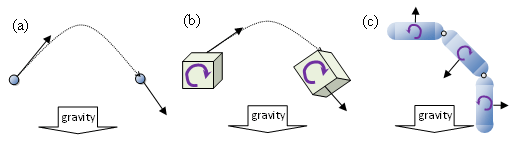Figure 1. Simple physical phenomena: (a) particles, (b) rigid bodies, and (c) articulated bodies

Deformable bodies can change shape but retain their connectedness and adjacency of various points on the body. Think of this as a model where the edges between vertices never change which vertices they connect, but the locations of the vertices can move. Their type depends on their dimensionality:

• 1D. Thread, string, rope, chain, hair, and so on, shown in Figure2(a)
• 2D. Cloth, as shown in Figure2(b)
• 3D. Soft bodies, like the jiggly bits of a character model, as shown in Figure2(c)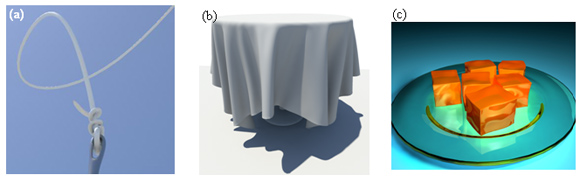Figure 2. Deformable bodies: (a) thread, (b) cloth and (c) soft bodies.

Fluids have lots of freedom of motion. The motion is nonlinear (more on that later), and their shape and topology can change, as shown in Figure 3. Fluids require specialized simulation techniques: Because fluids take the shape of their container, they are always in collision with everything around them, including the fluid itself. So a collision with one part of the fluid effectively means that the whole body of fluid must respond.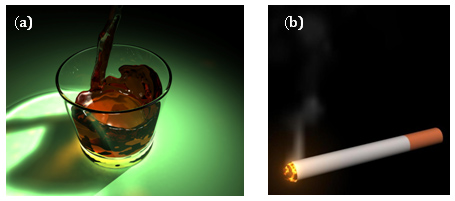Figure 3. Fluids: (a) liquid and (b) smoke.

### Representations and Coordinate Systems

You can model fluids in at least two ways: as a field, or as a collection of interacting particles. Both views are useful, and you often switch between them and combine them.

## Field-based Systems

To each point in a region containing fluid, you can ascribe a set of properties: velocity, density, temperature, and pressure. The positions of the points never move. This treatment of fluids as a field is called an Eulerian view. Figure 4(a) shows a simple case: a box of gas.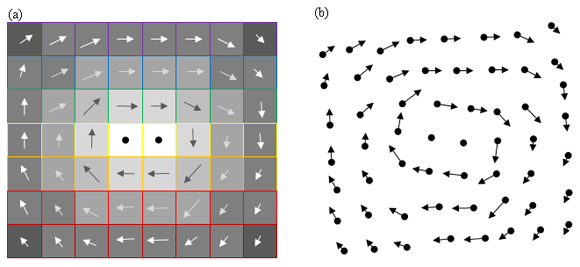Figure 4. Field-based and particle-based views of a fluid. (a) Grid based: Each point has fluid properties like velocity (arrows), density (box fill), pressure (arrow color), and temperature (box outline), and the grid points never move. (b) Particle based: Each particle has fluid properties in addition to position, and each particle can move.

## Particle-based Systems

You can also think of fluid in terms of a vast collection of particles (or parcels) that move around. Each parcel has properties such as position, velocity, density, and temperature. Note the addition of position here in contrast with the Eulerian view, where position is fixed to the grid. This treatment of fluids is called a Lagrangian view. Figure 4(b) shows a fluid as a collection of moving particles.

### Fluid Properties

At a microscopic level, fluids consist of a vast number of molecules whose principle interaction is collision. But the number of molecules is so large that you cannot pragmatically deal with them as such. Instead, you have to deal with them statistically, meaning that you pretend that clusters of particles act like a special substance that behaves differently than just a collection of particles. This special treatment entails (among other things) ascribing "bulk properties" to the fluid that characterize how the fluid interacts with itself.

The most common and important properties a fluid can have include these:

Pressure. Pressure refers to normal forces that apply to fluid parcels as well as the forces that fluid applies to its container and other solid objects embedded in the fluid, as Figure 5(a) shows.

Viscosity. Fluids also have shear forces, which act across the fluid, distorting it. Viscosity is the extent to which fluid resists that distortion, as Figure 5(b) shows. Thick fluids (like syrup) have high viscosity; thin fluids (like water) have low viscosity.

Density. Density expresses how much matter is in each small volume of space in the fluid.

Temperature. Temperature refers to how much heat resides in a fluid parcel. Temperature itself does not directly affect how the fluid moves, but it can affect pressure and density, which in turn affect motion.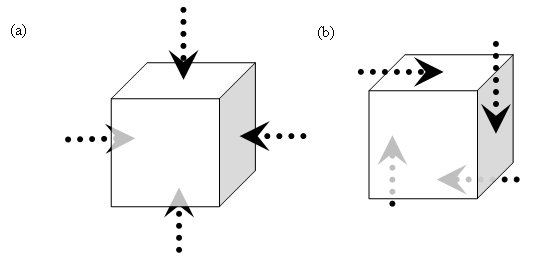Figure 5. Components of stress: (a) normal (pressure) and (b) shear

Fluids can have other, more sophisticated properties, such as more complex viscosity (for example, bouncing putty, blood or mucus) or composition (for example, fuel, oxygen, and exhaust), that you might want to include in your designs for more specialized fluid simulations (for example, combusting gas).

### Governing Equations

As with other physical phenomena like rigid bodies, systems of equations describe how a fluid evolves, or changes, over time. We call these equations governing equations. For a rigid body, the governing equations include Newton’s second law of motion, expressed as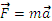, where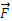is the force acting on the body, m is its mass, and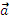is its acceleration -- that is, how its velocity changes direction and speed over time. Fluids are more complicated and have more than one set of governing equations. In addition, each set of equations has multiple forms, which can vary depending on what kind of fluid you want to model. An early step in modeling fluid motion entails choosing which governing equations to use. This article chooses relatively simple forms.

Modeling a fluid entails more than just its motion: You can also model its internal state (pressure, density, and temperature), heat transport, and other properties. This and the other articles in the series assume that temperature and density remain constant throughout the fluid; but remember that if you want to model more sophisticated fluid flows, you should explore concepts like the equation of state (for example, ideal gas law) and thermal diffusion (for example, Fourier heat conduction).

### Review of Partial Differentials

This section presents a very brief review of differential calculus.

First, some terminology: A scalar value has a single component, e.g. height. In contrast, a vector value has multiple components. For example, a 2-vector has 2 components (e.g. x and y) and a 3-vector has 3 components. A similar but distinct notion is the dimensionality of the function, which is how many variables the function depends on. So a 1D function is a function of a single variable, e.g. f(u), and a 2D function is a function of 2 variables, e.g. f(u,v). You can combine these notions. You're familiar with scalar function of 1 variable, often written f(x). But you can also have scalar functions of multiple variables (e.g. a single value is defined at every point on a 2D surface, such as a height-field) and vector functions of multiple variables (e.g. multiple values are defined at every point in a 3D volume, such as the components of velocity in a flow field).

You'll likely recall the notion that a derivative is the slope of a line tangent to a curve. You can extend that notion of a derivative to scalar and vector functions of higher dimension. The resulting operators include the gradient, divergence and curl, detailed below.

Recall that the derivative of a 1D scalar function (i.e. a function of a single variable, which has a single value) is the slope of the line tangent to the function at a given point, as shown in Fig. X(a). Likewise, the gradient of a scalar function of more variables is a combination of partial derivatives - one for each variable - combined to create a vector which points along the slope of that function, as Fig. X(b) depicts.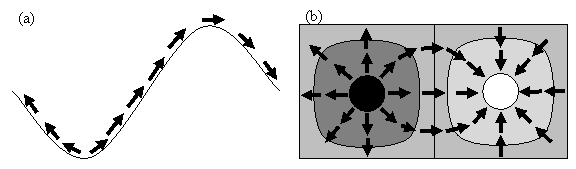Figure X: Derivatives of scalar functions.
(a) Ordinary derivative of a 1D scalar function is the slope of the line tangent to the curve. (b) Gradient of a 2D scalar function (e.g. terrain height) points up the slope of the "terrain".

## Divergence

The divergence of a vector function indicates how much of the field flows outward from a given point. Figure Y(a) shows a function that has divergence. Note that the divergence of a vector field is itself a scalar. If the vector field is a velocity field then a positive divergence implies the mass at the point decreases. Think of a tank of compressed gas emptying out; the volume of the container remains constant but the amount of gas inside the tank diminishes as gas flows outward.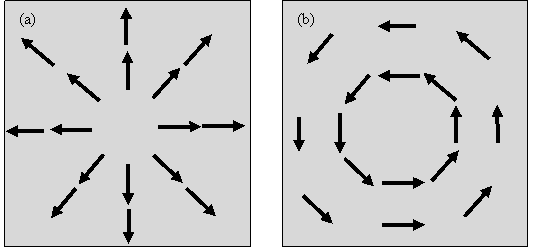Figure Y: Derivatives of vector functions.
(a) An irrotational vector field has only divergence (no curl). (b) A solenoidal vector field has only curl (no divergence).

## Curl

The curl of a vector field indicates the amount of circulation about each point. Figure Y(b) shows a vector field that has curl. The curl of a velocity field is called the vorticity. Note that the curl is itself a vector; to find its direction, we use the "right-hand rule": Curl the fingers of your right hand along the direction of the vectors and your thumb will point along the direction of the curl. In Fig. Y(b), the curl points out of the page

## Helmholtz Decomposition

The fundamental theorem of vector calculus states that you can represent a vector field as the sum of an irrotational part (which has no curl) and a solenoidal part (which has no divergence).

## Momentum

Consider Newton’s second law,, for a block. Remembering that acceleration is the rate of change of velocity -- that is,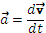, -- and dividing each side by mass, you can write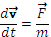. Now, imagine that block in contact with another block, as shown in Figure 6(b), and that the two blocks move relative to each other, as shown in Figure 6(c). Each block now has multiple forces acting on it: a normal force, a friction force, and a body force (gravity). Expanding the force term,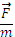, you get: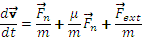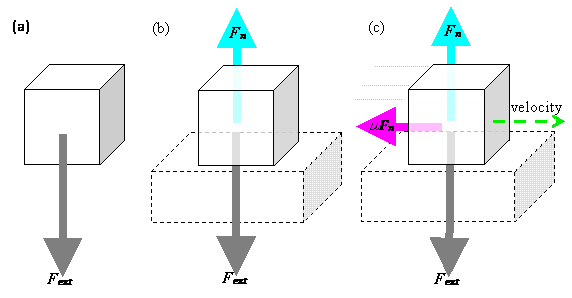Figure 6. Force diagrams for a block; (a) without contact, (b) resting contact, and (c) sliding contact

Similar to Newton's laws of motion, the Navier-Stokes equation expresses how velocity changes as a result of forces: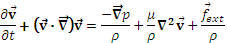Here,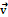is the velocity at a point in time and space, t is time, p is pressure at a point, p is the density of the fluid at a point, µ is viscosity, and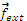are external forces, such as gravity, acting on the fluid.

Note the similarities and differences to the equation for the block. Both express how velocity changes over time. Both include forces resulting from contact as well as external forces. But the fluid equation has an extra term on the left,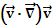, that takes some effort to understand.

The terms on the left express acceleration and have a special meaning. You can rewrite them as a new operator,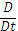: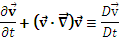This formula is called by many names, including the substantive derivative, advective derivative, Lagrangian derivative, and particle derivative. These names give a clue to its unusual meaning, and this is, in some sense, the heart of fluid motion. So, let's break it down, because to understand fluid motion, you must understand this derivative.

The term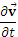expresses how fluid velocity at a fixed location changes over time. Note the qualifier "at a fixed location," which brings us back to the Eulerian view, shown in Figure 7, where you represent a fluid as a field and ask questions about how fluid properties at fixed locations in that field change over time. So, this term simply expresses the change in velocity over time (that is, the acceleration) of a point in a fluid field.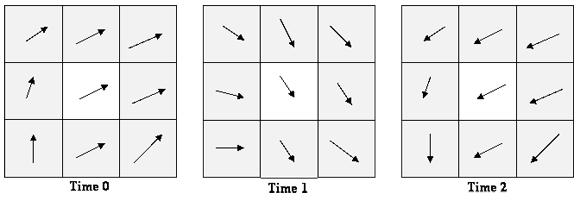Figure 7. Eulerian acceleration: velocity at a fixed location changes over time.

The termis tricky: It is called the advective term (see Figure 8) and expresses how velocity of a fluid parcel changes as that fluid parcel moves around—basically, velocity changes as a result of moving around in a velocity field. Again imagine that a fluid is a field in which every point in the field has a velocity. This would be like walking around in an airport that has slidewalks (moving sidewalks) everywhere. And these are unusual slidewalks that move in different directions and different speeds at different places, but the direction and speed remain the same at each location. Imagine wandering around in this crazy airport: Depending on where you stood, the slidewalks would carry you in different directions and speeds. By standing—without walking—on this crazy network of slidewalks, you would change speed and direction. You would accelerate simply as a result of following the flow field.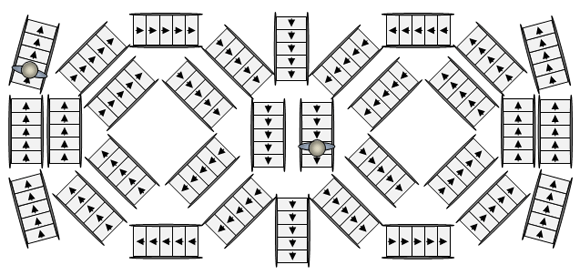Figure 8. Advective acceleration: Velocity at each point remains constant, but a tracer following the field causes the tracer to accelerate.

Notice that the advective term,, has velocity in it twice: That repetition makes the motion nonlinear. When people refer to the nonlinear motion of fluids, they refer to this term -- advective acceleration. This term is the main reason why fluids have such complicated motion. When writing a simulation, a good deal of your effort goes into handling this term.

When you combine these two terms, you ask how a fluid parcel accelerates both as it follows the flow field and as a result of the flow field itself changing in time. When you ask about these together, you adopt the Lagrangian view -- that is, you think of the fluid as a collection of particles. So, the difference between Eulerian and Lagrangian views effectively boils down to where you put the advective term,: on the right side or on the left side of the momentum equation.

## Mass

When pressure applies to a parcel of fluid, the fluid can compress or expand. You express this compression or expansion mathematically simply by stating that an influx of fluid changes the amount of fluid at that location: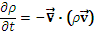For visual effects, you can usually neglect compressibility, so simplify this equation to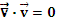. In that case, the pressure becomes coupled to velocity and we can drop the pressure term from the momentum equation (but as we will see in the second article, pressure reappears in another form). Any vector field with zero divergence is called "solenoidal". This condition ends up causing some complication in fluid simulations, which the second article in this series will revisit.

Mass can also advect and diffuse, in which case the form of its governing equations resemble the momentum equation given above, except without the pressure term. In other words, density follows the flow and diffuses.

## Vorticity

You can readily imagine a vortex or multiple vortices, because they appeal to intuition. Anybody who has watched a tornado, water flowing down a drain, or milk stirred into coffee has seen vortices. Smoke rings are also really just vortex rings-vortices that loop back on themselves. In fluid dynamics, we call these loops coherent structures, because they seem to have a persistent life of their own. The "vorticity" equation describes how these structures evolve.

Vorticity,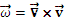(the curl of velocity) describes how fluid rotates. By taking the curl of the momentum equation, you derive the vorticity equation: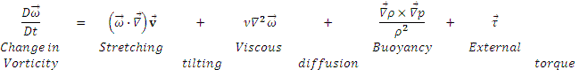By solving this equation, you can obtain a complete description of fluid motion. Vorticity gives fluid its characteristic swirling motion. Figure 9 shows examples of simple vortical flows.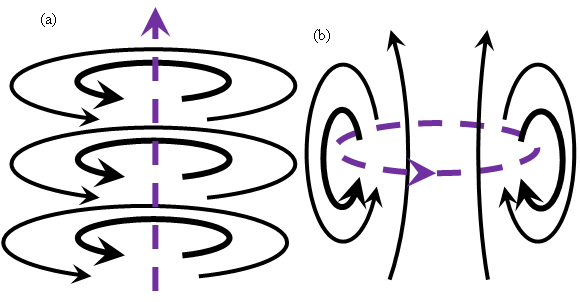Figure 9. Vortices and their flows: (a) a line vortex (purple dashed line) and the circular flow around it (black solid line), and (b) a ring vortex and the "jet" flow through it

The stress term,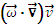, describes the stretching and tilting of vortices, as shown in Figure 10. Vortex stretching is an important process in the turbulent cascade of energy from larger to smaller scales in the flow and only occurs in 3D flows.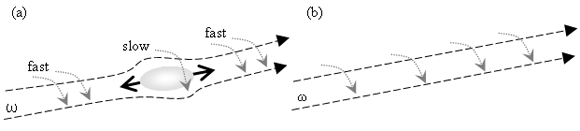Figure 10. Vortex stretching: (a) A vortex tube with a bulge with velocity flowing outward from the bulge causes the bulge to shrink. (b) After the bulge squirted away, the tube shrank: The amount of mass rotating there decreased, so to conserve angular momentum, vorticity increased. In other words, the tube rotates faster where it initially rotated more slowly.

The viscous term,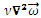, describes the diffusion of vorticity—that is, how vorticity spreads as a result of friction.

The final term expresses buoyancy, which (as shown in Figure 11) creates regions of overturning, where fluids with density out of equilibrium (for example, heavy fluid above light fluid) form rolling currents that tend toward bringing the fluid into equilibrium (that is, putting heavy fluid below light fluid).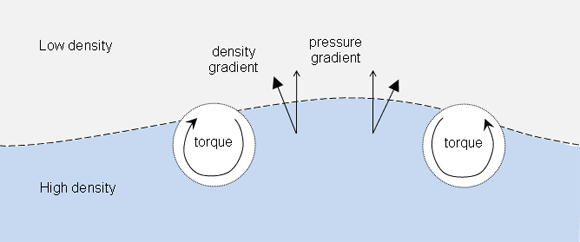Figure 11. Keeping fluid right-side up. Where the pressure gradient and the density gradient are not parallel, vorticity forms to bring fluid layers into equilibrium. Fluid will try to rotate to push down the bulge.

The momentum equation uses pressure, so this approach to solving the fluid dynamics equations is sometimes called the velocity-pressure formulation, or primitive variable formulation. In contrast, the vorticity equation does not require pressure, but it does require velocity, so this approach to solving the fluid dynamics equations is sometimes called the vorticity-velocity formulation.

The vorticity equation is redundant with the momentum equation: You only need to solve one or the other, as they are equivalent. Once you get used to the idea of vorticity, it becomes easier to work with than momentum, especially because you can readily and intuitively identify a vortex and follow its motion.

### Boundary Conditions

Fluids interact with their containers, with objects embedded in the fluid, and with other, distinct fluids that do not mix (for example, air and water). You express these interactions as boundary conditions, which have two components:

No-through. Fluid cannot flow into or out of a body.

No-slip. Fluid cannot move across a body. (Alternatively, you can use free-slip boundaries, which are not perfectly realistic but apply for fluids without viscosity.)

Boundary conditions express how a body influences fluid flow as well as how fluid flow influences the motion of a body. This problem has multiple solutions. For example, you can ask about the pressure field on the boundary or use conservation laws (like linear and angular momentum) to exchange impulses between the fluid and the body.

### Summary

Adding fluid simulations to video games can make the games more immersive and compelling. This article introduced fluid dynamics properties and equations in preparation for a description of algorithms used to simulate fluid motion. Because it includes more degrees of freedom and nonlinear motion, fluid dynamics is more complicated than other, familiar forms of physical phenomena such as rigid body dynamics. Fluid dynamics employs partial differential equations (in contrast to ordinary differential equations) and has boundary conditions (in addition to initial conditions). Simulation techniques suitable for fluids have a corresponding delicacy and complexity that subsequent articles in this series will explore. The next article will survey fluid simulation techniques, including grid-based, grid-free, and hybrid methods. The final article will provide a vortex particle fluid simulation, which you can use to augment an existing particle system in a game.

### Latest Jobs

#### Treyarch

5.8.23
Producer

Remote (United States)
5.18.23
Senior Gameplay Engineer

#### University of Canterbury

Christchurch, Canterbury, New Zealand
5.17.23
Academic in Game Arts and Animation

#### Fred Rogers Productions

Hybrid (424 South 27th Street, Pittsburgh, PA, USA
5.19.23
Producer - Games & Websites
More Jobs

#### Game Developer Job Board

Browse open positions across the game industry or recruit new talent for your studio